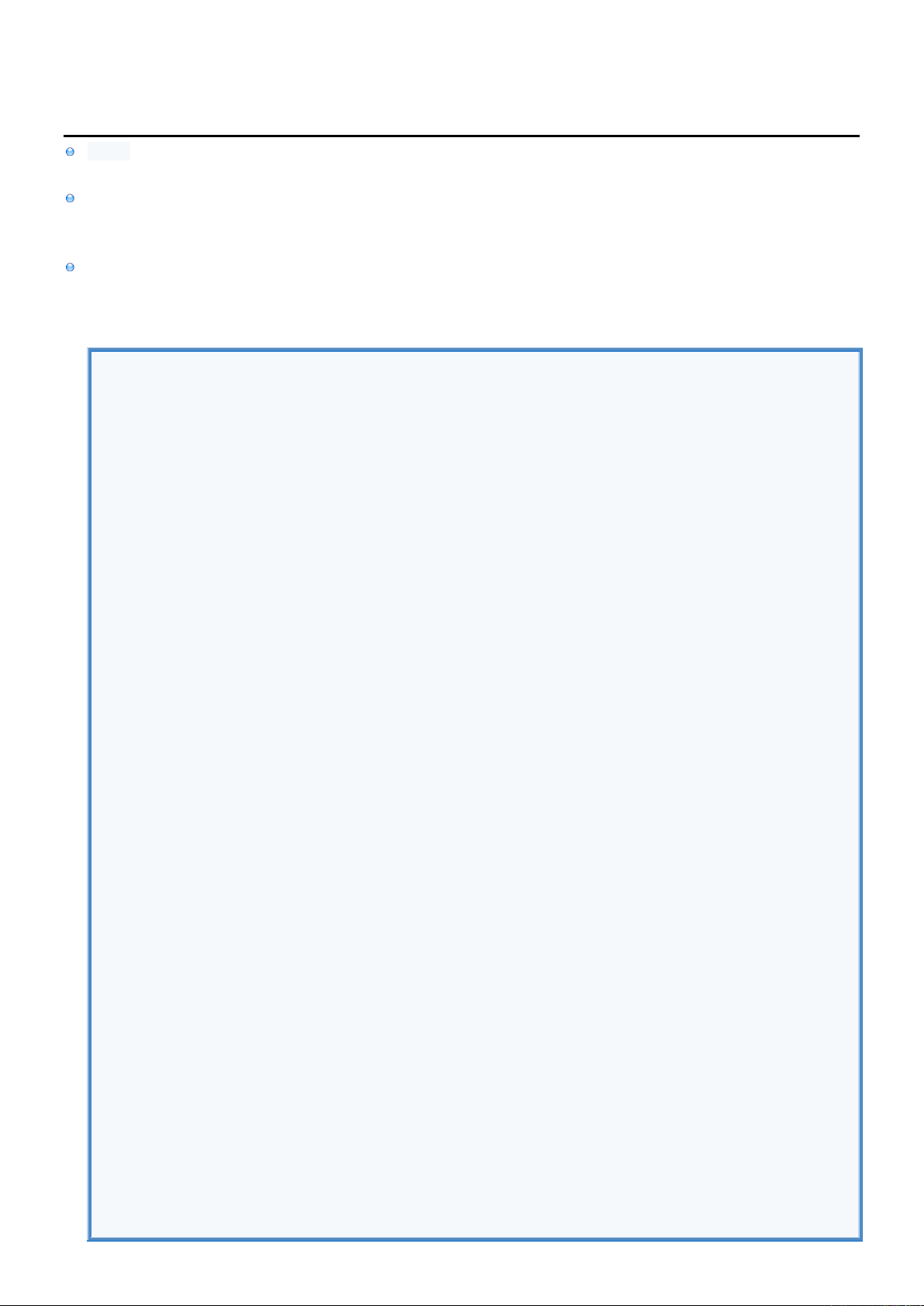2
2
.
.
6
6
.
.
4
4
V
V
e
e
c
c
t
t
o
o
r
r
I
I
n
n
f
f
o
o
Vector is variable collection of indexed elements.
Index is integer starting with 0 and can't be negative.
Elements can only be inserted at the end or at the already existing index.
Existing element at that index and all elements with bigger indexes are shifted to the right by increasing their index by 1
Size of vector is increased by 1.
When element is removed all elements with bigger indexes are shifted to the left by decreasing their index by 1.
Size of vector is decreased by 1.
Test.java
import java.util.Vector;
public class Test {
public static void main (String arg[]) throws Exception {
//PUT ELEMENTS.-------------------------------------
Vector vector = new Vector();
vector.addElement( "element" ); //Append element to the end of vector.
vector.add ( 33 ); //Append element to the end of vector.
vector.add ( false ); //Elements can be of different Raw Type
vector.add ( new Character('A')); //or Object.
vector.add (3, "Shift others" ); //Insert element at index 3 and add 1 to
//indexes of elements on index 3 or above.
//GET ELEMENTS.-------------------------------------
String index_0 = (String ) vector.elementAt(0);
int index_1 = ( (Integer ) vector.get (1)).intValue ();
boolean index_2 = ( (Boolean ) vector.get (2)).booleanValue();
String index_3 = (String ) vector.get (3);
char index_4 = ( (Character) vector.get (4)).charValue ();
//STATISTICS.---------------------------------------
boolean containsElement = vector.contains(33);
boolean isEmpty = vector.isEmpty ( );
int size = vector.size ( );
String removed = (String) vector.remove (3 ); //Decrase size & indexes of above elements by 1
vector.clear ( );
//DISPLAY ELEMENTS.---------------------------------
Iterator iterator = vector.iterator();
while( iterator.hasNext() == true ) {
System.out.println(iterator.next());
iterator.remove();
}
//DISPLAY STATISTICS.-------------------------------
System.out.println ("Contains 'element' = " + containsElement);
System.out.println ("isEmpty = " + isEmpty );
System.out.println ("size = " + size );
System.out.println ("removed element = " + removed );
}
}